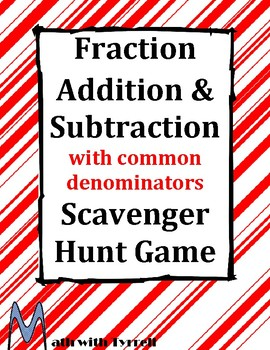# Fraction Addition & Subtraction with Common Denominators Scavenger Hunt GameSubject
Resource Type
File Type

PDF

(548 KB|38 pages)
Standards
Also included in:
1. Scavenger hunts are an excellent activity to get students moving around the classroom while being engaged with the mathematics. In these activities, students use the answer to one problem to find another problem hanging around the classroom. The student worksheets, scavenger hunt problems, instruct
\$21.00
\$18.90
Save \$2.10
• Product Description
• Standards

Do you need a fun way for students to practice adding and subtracting fractions with minimal prep? The Fraction Addition and Subtraction with Common Denominators Scavenger Hunt Game gets students up and moving around while practicing math. In this activity, students use the answer to one problem to find another problem hanging around the classroom. Students will complete a total of 16 problems to create a list of bones. This product is self-checking and allows a teacher to work one-on-one with students while the rest of the class is engaged in the scavenger hunt. My students love seeing scavenger hunts on the agenda. This product comes with the student worksheet, scavenger hunt problems, instructions, and teacher’s key. You can find a free video showing how I introduce a scavenger hunt to my students. Feel free to use this video in your classroom or use it to prepare your lesson.

This scavenger hunt deals with positive fractions only.

This product is also included in the Middle School Scavenger Hunt Bundle and Fraction Scavenger Hunt Bundle.

You may also be interested in:

Reducing Fractions Scavenger Hunt Game

Fraction Addition and Subtraction with Common Denominators Scavenger Hunt Game

Fraction Addition and Subtraction with Positives and Negatives Scavenger Hunt Game

Fraction Multiplication and Division Positives Only Scavenger Hunt Game

Fraction Multiplication and Division with Positives and Negatives Scavenger Hunt Game

Fraction and Mixed Numbers Operations Scavenger Hunt Game

Decimal and Fraction Addition and Subtraction Scavenger Hunt Game: positive fractions and decimals only

Decimal and Fraction Multiplication and Division Scavenger Hunt Game: positive fractions and decimals only

Thank you to Tracee Orman for the awesome clipart.

Add and subtract fractions with unlike denominators (including mixed numbers) by replacing given fractions with equivalent fractions in such a way as to produce an equivalent sum or difference of fractions with like denominators. For example, 2/3 + 5/4 = 8/12 + 15/12 = 23/12. (In general, 𝘢/𝘣 + 𝘤/𝘥 = (𝘢𝘥 + 𝘣𝘤)/𝘣𝘥.)
Add and subtract mixed numbers with like denominators, e.g., by replacing each mixed number with an equivalent fraction, and/or by using properties of operations and the relationship between addition and subtraction.
Understand addition and subtraction of fractions as joining and separating parts referring to the same whole.
Total Pages
38 pages
Included
Teaching Duration
40 minutes
Report this Resource to TpT
Reported resources will be reviewed by our team. Report this resource to let us know if this resource violates TpT’s content guidelines.